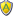[ New messages · Members · Forum rules · Search · RSS ]
 Forum » General category » General forum » Jim Klutho Basic (experimental basic)
Jim Klutho Basic
 Zlatko Date: Sunday, 2017-05-28, 6:52 PM | Message # 1 LieutenantGroup: Administrators Messages: 62 Reputation: 0 Status: Offline in attchment is zipped version Attachments: JimK3.o2bas(27.0 Kb)
 Zlatko Date: Sunday, 2017-05-28, 6:53 PM | Message # 2 LieutenantGroup: Administrators Messages: 62 Reputation: 0 Status: Offline Here is image of AurelEditAttachments: 2649936.png(108.8 Kb)
 Zlatko Date: Sunday, 2017-05-28, 7:00 PM | Message # 3 LieutenantGroup: Administrators Messages: 62 Reputation: 0 Status: Offline This is improved TOKENIZER'tokenizer aka lexer! IsAlpha    (byval in as string) as int! IsDigit    (byval in as string) as int! IsEquate   (byval in as string) as int! IsWhite    (byval in as string) as int! IsComma    (byval in as string) as int! IsDelimiter(byval in as string) as int! IsPlus     (byval in as string) as int! IsMinus    (byval in as string) as int! IsMulti    (byval in as string) as int! IsDevide   (byval in as string) as int'global vars...string input ,ch ,nextch, tokBuff, pchstring CRLF = chr(13)+chr(10)dim pos as intdim inputLen as intINT TRUE = 1 ,FALSE = 0INT tokpos=1String token,tokList' input stringinput = "def a,b ,a2, b=7,c+5 ,d=3.14 " inputLen = Len(input)                   ' string lengthpos = 1                                 ' start position'loop trough input string reading each char >>>WHILE pos <= inputLench=""ch = mid input,pos,1        'current charnextch = mid input,pos+1,1  'next char    'test if is letter    If IsAlpha(ch) = TRUE         'print ch + " : IS Alpha"    token = token + ch ' buffering token string    End if    'test if is number    If IsDigit(ch) = TRUE OR ch="."        'print ch + " : IS Digit"    token = token + ch ' buffering token string    End if   '/// if is end of token/ is delimiter ` `,`+ ` -` *`/`%'push alphanum token into tokenListIF isDelimiter(nextCh)=TRUE AND ( IsAlpha(ch)=TRUE OR IsDigit(ch)=TRUE )       tokList [tokpos]= token           ' push token into tokenList    tokBuff = tokBuff & token  & CRLF ' push token to tokenBuffer    tokpos=tokpos+1 : token = ""   'end ifEND IFIf isDelimiter(ch) = TRUE    If isEquate(ch)=TRUE  ' sym =     token = ch     tokList [tokpos]= token               tokBuff = tokBuff & token  & CRLF     tokpos=tokpos+1 : token = ""    End if    If isPlus(ch)=TRUE  ' sym +     token = ch     tokList [tokpos]= token               tokBuff = tokBuff & token  & CRLF     tokpos=tokpos+1 : token = ""    End if    If isMinus(ch)=TRUE  ' sym -     token = ch     tokList [tokpos]= token               tokBuff = tokBuff & token  & CRLF     tokpos=tokpos+1 : token = ""    End if    If isMulti(ch)=TRUE  ' sym *     token = ch     tokList [tokpos]= token              tokBuff = tokBuff & token  & CRLF    tokpos=tokpos+1 : token = ""    End if    If isDevide(ch)=TRUE  ' sym /     token = ch     tokList [tokpos]= token               tokBuff = tokBuff & token  & CRLF     tokpos=tokpos+1 : token = ""    End ifEnd if'if comment then exit loopIf nextch="'" then EXIT WHILE pch = ch ' set p-last char to current charpos=pos+1 'move to next char in input string Wend'<<<'show token bufferprint tokBuff ' /// LEXER SUBROTINES ////////////////////Sub IsAlpha(string s) as intint asci : asci = asc(s)    select asci        case 65 to 90 'A->Z         return TRUE         case 97 to 122 'a->z         return TRUE    end selectreturn FALSEEnd SubSub IsDigit(string s) as INTint asci : asci = asc(s)    select asci        case 48 to 57 '0->9         return TRUE    end selectreturn FALSEEnd SubSub isDelimiter(string s) as INTINT chPoschPos = INSTR(" ,+-*/^%()<>=:",s)If chPos > 0    Return TRUEEnd IfReturn FALSEEnd SubSub IsWhite(string s) as INTint asci: asci = asc(s)    select asci        case 32 ' " "->empty space         return TRUE         case 0         return TRUE    end selectreturn FALSEEnd SubSub IsComma(string s) as INTint asci: asci = asc(s)    select asci        case 44 ' ","-> comma         return TRUE    end selectreturn FALSEEnd SubSub IsEquate(string s) as INTint asci: asci = asc(s)    select asci        case 61 ' "="-> equal         return TRUE    end selectreturn FALSEEnd SubSub IsPlus(string s) as INTint asci: asci = asc(s)    select asci        case 43 ' "+"-> plus         return TRUE    end selectreturn FALSEEnd SubSub IsMinus(string s) as INTint asci: asci = asc(s)    select asci        case 45 ' "-"-> minus         return TRUE    end selectreturn FALSEEnd SubSub IsMulti(string s) as INTint asci: asci = asc(s)    select asci        case 42 ' "*"-> multiply         return TRUE    end selectreturn FALSEEnd SubSub IsDevide(string s) as INTint asci: asci = asc(s)    select asci        case 47 ' "/"-> devide         return TRUE    end selectreturn FALSEEnd Sub
 Forum » General category » General forum » Jim Klutho Basic (experimental basic)
• Page 1 of 1
• 1
 Search: